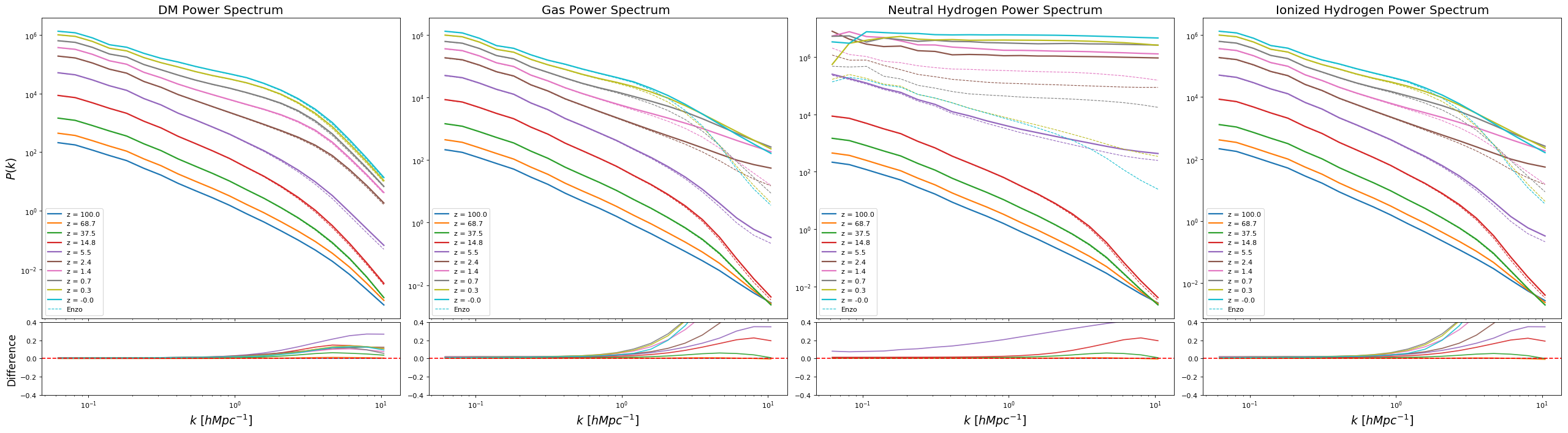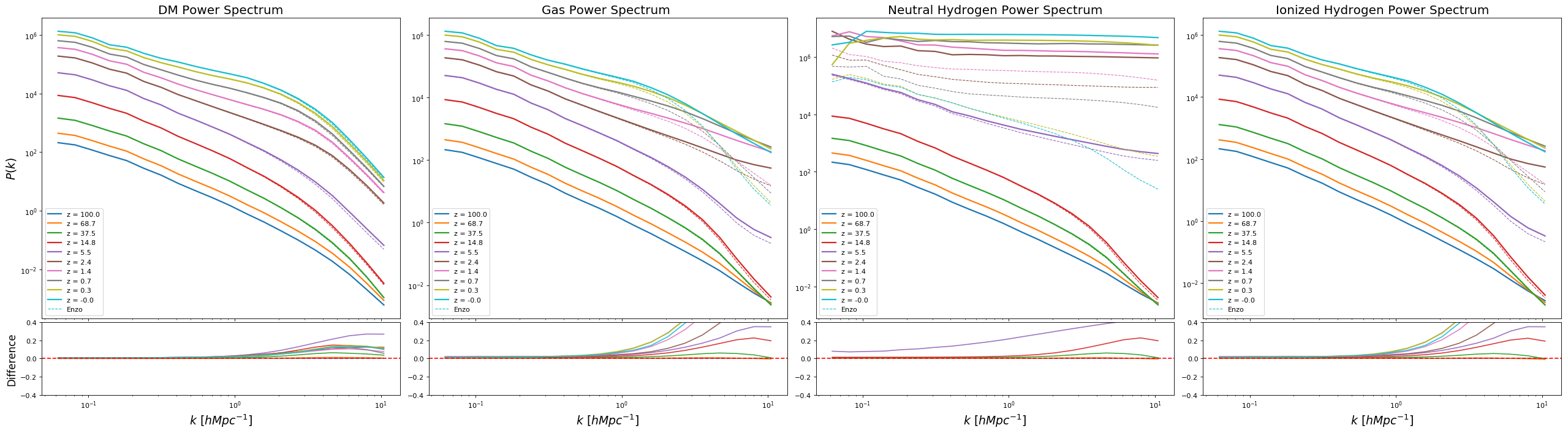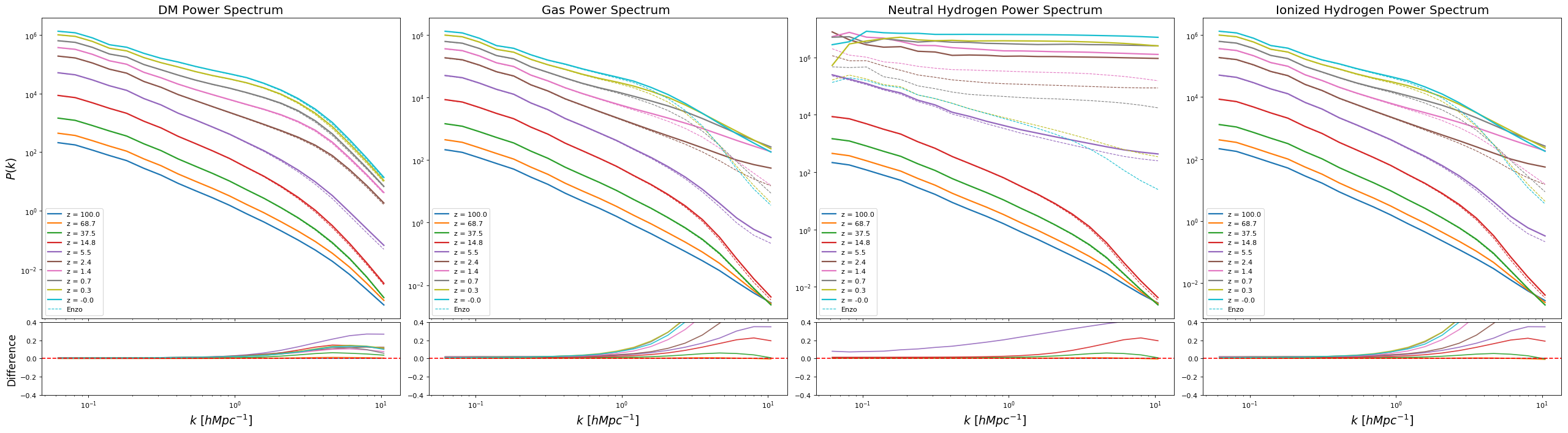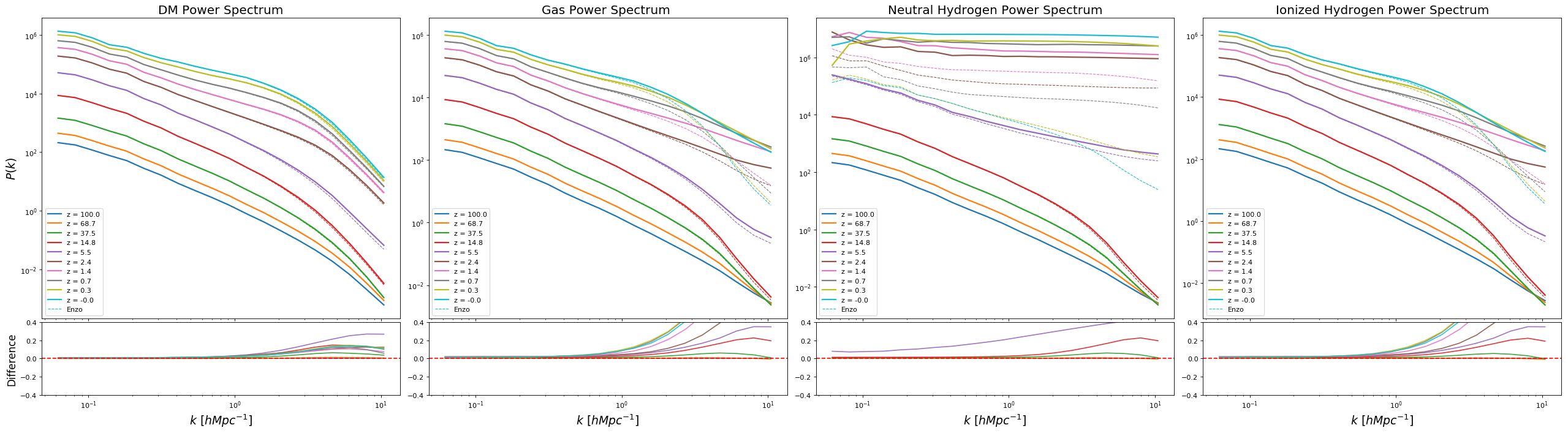Here is the result of the cosmological simulations using the Enzo and Teyssier conditions for dual energy.

Simulation using PPMC + HLLC + SIMPLE, and $\eta=0.001$

## Phase Diagram

Row 1: $\beta = 0.3$ Row 2: $\beta = 0.5$ Row 3: $\beta = 0.7$ Row 4: $\beta = 0.9$

## Power Spectrum

1: $\beta = 0.3$2: $\beta = 0.5$3: $\beta = 0.7$4: $\beta = 0.9$